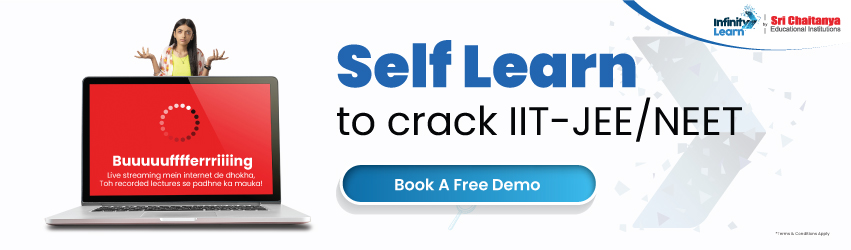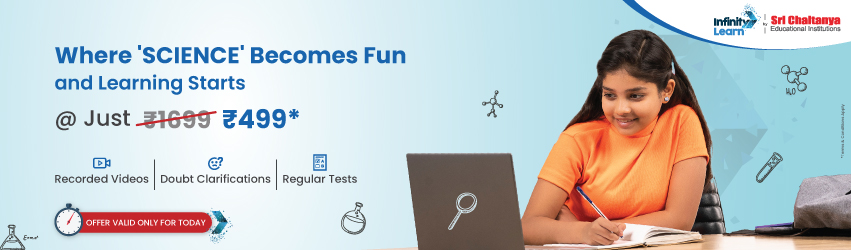RD Sharma Class 12 Solutions Chapter 23 Algebra of Vectors Ex 23.2

# RD Sharma Class 12 Solutions Chapter 23 Algebra of Vectors Ex 23.2infinitylearn surge self learn
infinitylearn starter package

## RD Sharma Class 12 Solutions Chapter 23 Algebra of Vectors Ex 23.2

Algebra of Vectors Ex 23.2 Q1Algebra of Vectors Ex 23.2 Q2Algebra of Vectors Ex 23.2 Q3Algebra of Vectors Ex 23.2 Q4Algebra of Vectors Ex 23.2 Q5Algebra of Vectors Ex 23.2 Q6Algebra of Vectors Ex 23.2 Q7Algebra of Vectors Ex 23.2 Q8Algebra of Vectors Ex 23.2 Q9Algebra of Vectors Ex 23.2 Q10Need FREE NCERT/CBSE/IIT-JEE/NEET Study Material?